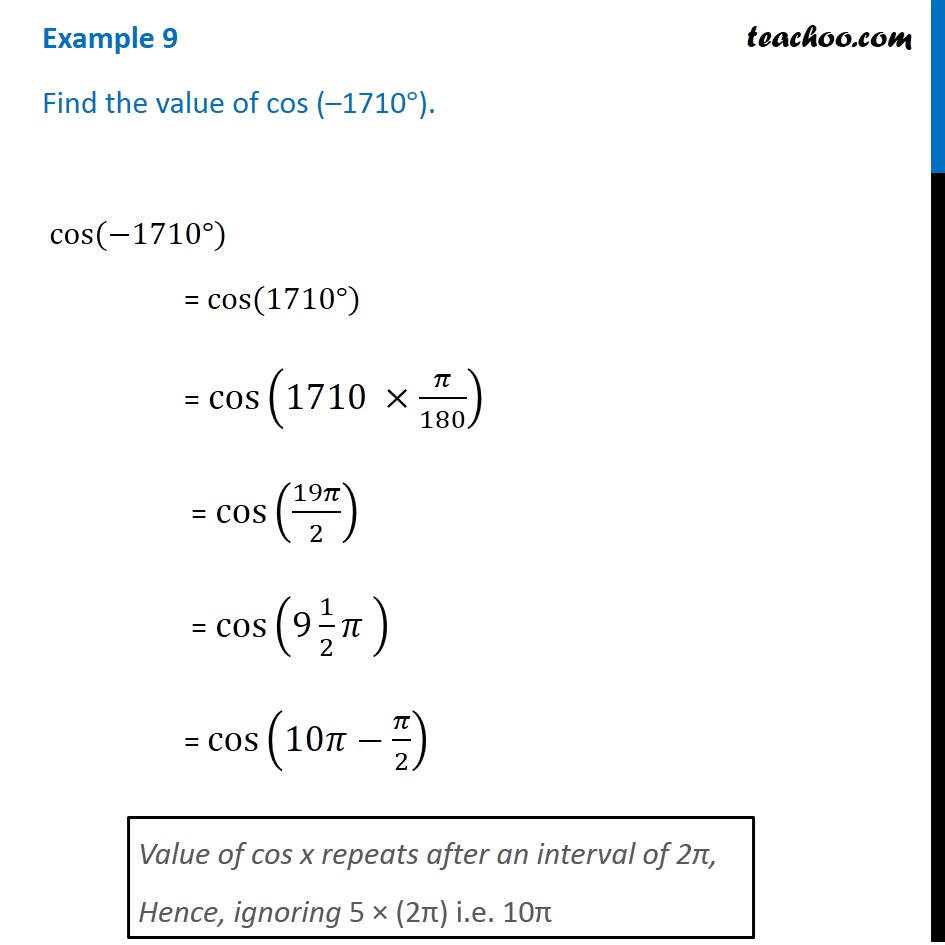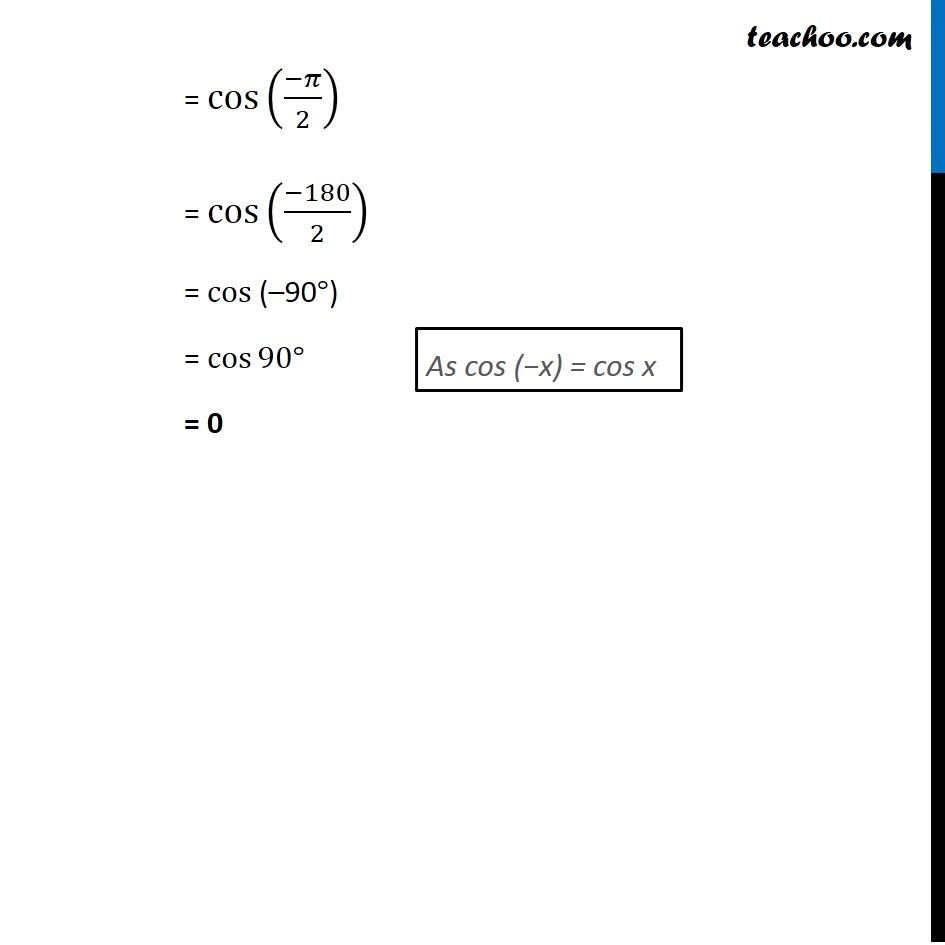Learn All Concepts of Chapter 2 Class 11 Relations and Function - FREE. Check - Trigonometry Class 11 - All Concepts1. Chapter 3 Class 11 Trigonometric Functions
2. Concept wise
3. Finding Value of trignometric functions, given angle

Transcript

Example 9 Find the value of cos (–1710°). cos⁡(−1710°) = cos⁡(1710°) = cos⁡(1710 ×𝜋/180) = cos⁡(19𝜋/2) = cos⁡(9 1/2 𝜋 ) = cos⁡(10𝜋−𝜋/2) Value of cos x repeats after an interval of 2π, Hence, ignoring 5 × (2π) i.e. 10π = cos⁡((−𝜋)/2) = cos⁡((−180)/2) = cos⁡(–90°) = cos⁡90° = 0 As cos (−x) = cos x

Finding Value of trignometric functions, given angle

About the AuthorDavneet Singh
Davneet Singh is a graduate from Indian Institute of Technology, Kanpur. He has been teaching from the past 9 years. He provides courses for Maths and Science at Teachoo.